1. /
2. CBSE
3. /
4. Class 08
5. /
6. Mathematics
7. /
8. NCERT Solutions for Class...

# NCERT Solutions for Class 8 Maths Exercise 15.2### myCBSEguide App

Download the app to get CBSE Sample Papers 2023-24, NCERT Solutions (Revised), Most Important Questions, Previous Year Question Bank, Mock Tests, and Detailed Notes.

NCERT solutions for Class 8 Maths Introduction to Graphs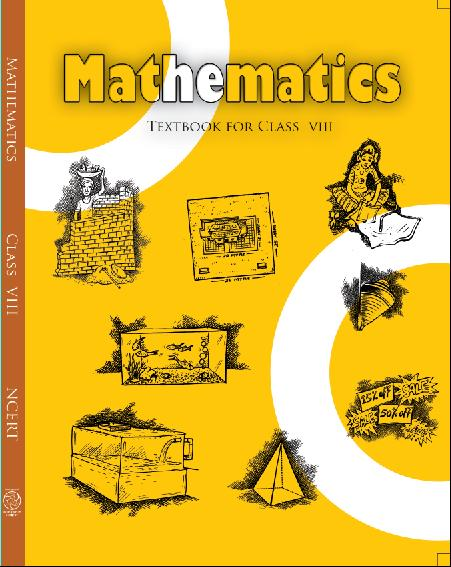## NCERT Solutions for Class 8 Maths Introduction to Graphs

###### 1. Plot the following points on a graph sheet. Verify if they lie on a line

(a) A(4,0), B(4,2), C(4,6), D(4, 2.5)

(b) P(1,1), Q(2,2), R(3,3), S(4,4)

(c) K(2, 3), L(5, 3), M(5, 5), N(2, 5)

Ans.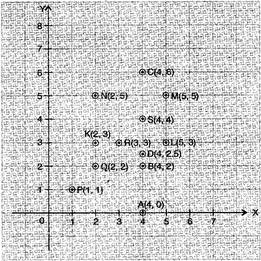(a) All points A, B, C and D lie on a vertical line.

(b) P, Q, R and S points also make a line. It verifies that these points lie on a line.

(c) These points do not lie in a straight line.

###### 2. Draw the line passing through (2, 3) and (3, 2). Find the coordinates of the points at which this line meets the x-axis and y-axis.

Ans.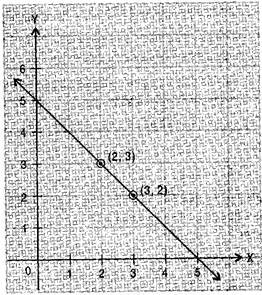The coordinates of the points at which this line meets the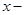axis at (5, 0) and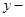axis at (0, 5).

NCERT Solutions for Class 8 Maths Exercise 15.2

###### 3. Write the coordinates of the vertices of each of these adjoining figures.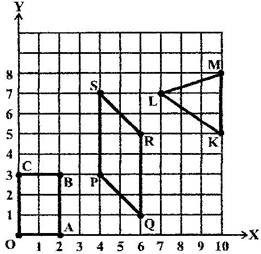Ans. Vertices of figure OABC

O (0, 0), A (2, 0), B (2, 3) and C (0, 3)

Vertices of figure PQRS

P (4, 3), Q (6, 1), R (6, 5) and S (4, 7)

Vertices of figure LMK

L (7, 7), M (10, 8) and K (10, 5)

NCERT Solutions for Class 8 Maths Exercise 15.2

###### 4. State whether True or False. Correct that are false.

(i) A point whose x coordinate is zero and y-coordinate is non-zero will lie on the y-axis.

(ii) A point whose y coordinate is zero and x-coordinate is 5 will lie on y-axis.

(iii) The coordinates of the origin are (0, 0).

Ans. (i) True (ii) False, it will lie onaxis. (iii) True

## NCERT Solutions for Class 8 Maths Exercise 15.2

NCERT Solutions Class 8 Mathematics PDF (Download) Free from myCBSEguide app and myCBSEguide website. Ncert solution class 8 Mathematics includes text book solutions from Class 8 Maths Book . NCERT Solutions for CBSE Class 8 Maths have total 16 chapters. 8 Maths NCERT Solutions in PDF for free Download on our website. Ncert class 8 solutions PDF and Maths ncert class 8 PDF solutions with latest modifications and as per the latest CBSE syllabus are only available in myCBSEguide.

## CBSE app for Class 8

To download NCERT Solutions for class 8 Social Science, Computer Science, Home Science,Hindi ,English, Maths Science do check myCBSEguide app or website. myCBSEguide provides sample papers with solution, test papers for chapter-wise practice, NCERT solutions, NCERT Exemplar solutions, quick revision notes for ready reference, CBSE guess papers and CBSE important question papers. Sample Paper all are made available through the best app for CBSE students and myCBSEguide website.Test Generator

Create question paper PDF and online tests with your own name & logo in minutes.myCBSEguide

Question Bank, Mock Tests, Exam Papers, NCERT Solutions, Sample Papers, Notes

### 4 thoughts on “NCERT Solutions for Class 8 Maths Exercise 15.2”

1. my favourite site

2. My Favourite Site

3. Helped me in my studies